Download Presentation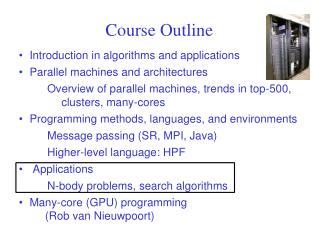Course Outline

# Course Outline - PowerPoint PPT Presentation

Course Outline. Introduction in algorithms and applications Parallel machines and architectures Overview of parallel machines, trends in top-500, clusters, many-cores Programming methods, languages, and environments Message passing (SR, MPI, Java) Higher-level language: HPFI am the owner, or an agent authorized to act on behalf of the owner, of the copyrighted work described.
Download Presentation## Course Outline

An Image/Link below is provided (as is) to download presentation

Download Policy: Content on the Website is provided to you AS IS for your information and personal use and may not be sold / licensed / shared on other websites without getting consent from its author.While downloading, if for some reason you are not able to download a presentation, the publisher may have deleted the file from their server.

- - - - - - - - - - - - - - - - - - - - - - - - - - E N D - - - - - - - - - - - - - - - - - - - - - - - - - -
Presentation Transcript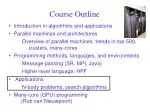Course Outline

Introduction in algorithms and applications

Parallel machines and architectures

Overview of parallel machines, trends in top-500, clusters, many-cores

Programming methods, languages, and environments

Message passing (SR, MPI, Java)

Higher-level language: HPF

Applications

N-body problems, search algorithms

Many-core (GPU) programming (Rob van Nieuwpoort)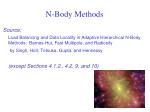N-Body Methods

Source:

Load Balancing and Data Locality in Adaptive Hierarchical N-Body Methods: Barnes-Hut, Fast Multipole, and Radiosity

by Singh, Holt, Totsuka, Gupta, and Hennessy

(except Sections 4.1.2., 4.2, 9, and 10)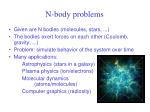N-body problems
• Given are N bodies (molecules, stars, ...)
• The bodies exert forces on each other (Coulomb, gravity, ...)
• Problem: simulate behavior of the system over time
• Many applications:

Astrophysics (stars in a galaxy)

Plasma physics (ion/electrons)

Molecular dynamics (atoms/molecules)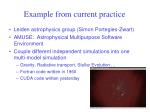Example from current practice

Leiden astrophysics group (Simon Portegies-Zwart)

AMUSE: Astrophysical Multipurpose Software Environment

Couple different independent simulations into one multi-model simulation

Gravity, Radiative transport, Stellar Evolution ...

Fortran code written in 1960

CUDA code written yesterday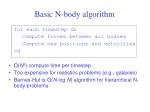Basic N-body algorithm

for each timestep do

Compute forces between all bodies

Compute new positions and velocities

od

• O(N2) compute time per timestep
• Too expensive for realistics problems (e.g., galaxies)
• Barnes-Hut is O(N log N) algorithm for hierarchical N-body problems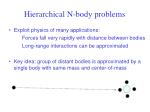Hierarchical N-body problems
• Exploit physics of many applications:

Forces fall very rapidly with distance between bodies

Long-range interactions can be approximated

• Key idea: group of distant bodies is approximated by a single body with same mass and center-of-mass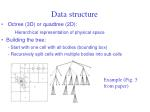Data structure
• Octree (3D) or quadtree (2D):

Hierarchical representation of physical space

• Building the tree:

- Start with one cell with all bodies (bounding box)

- Recursively split cells with multiple bodies into sub-cells

Example (Fig. 5 from paper)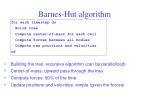Barnes-Hut algorithm

for each timestep do

Build tree

Compute center-of-mass for each cell

Compute forces between all bodies

Compute new positions and velocities

od

• Building the tree: recursive algorithm (can be parallelized)
• Center-of-mass: upward pass through the tree
• Compute forces: 90% of the time
• Update positions and velocities: simple (given the forces)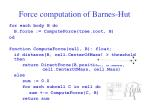Force computation of Barnes-Hut

for each body B do

B.force := ComputeForce(tree.root, B)

od

function ComputeForce(cell, B): float;

if distance(B, cell.CenterOfMass) > threshold then

return DirectForce(B.position, B.Mass, cell.CenterOfMass, cell.Mass)

else

sum := 0.0

for each subcell C in cell do

sum +:= ComputeForce(C, B)

return sum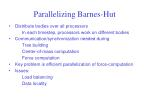Parallelizing Barnes-Hut
• Distribute bodies over all processors

In each timestep, processors work on different bodies

• Communication/synchronization needed during

Tree building

Center-of-mass computation

Force computation

• Key problem is efficient parallelization of force-computation
• Issues:

Data locality• Goal:

Each processor must get same amount of work

• Problem:

Amount of work per body differs widelyData locality
• Goal:

- Each CPU must access a few bodies many times

- Reduces communication overhead

• Problems

- Access patterns to bodies not known in advance

- Distribution of bodies in space changes (slowly)Simple distribution strategies
• Distribute iterations

Iteration = computations on 1 body in 1 timestep

• Strategy-1: Static distribution

Each processor gets equal number of iterations

• Strategy-2: Dynamic distribution

Distribute iterations dynamically

• Problems
• Distributing iterations does not take locality into account
• Static distribution leads to load imbalancesMore advanced distribution strategies
• Load balancing: cost model

Associate a computational cost with each body

Cost = amount of work (# interactions)in previous timestep

Each processor gets same total cost

Works well, because system changes slowly

• Data locality: costzones

Observation: octree more or less represents spatial (physical) distribution of bodies

Thus: partition the tree, not the iterations

Costzone: contiguous zone of costsExample costzones

Optimization:

improve locality using clever child numbering schemeExperimental system- DASH
• DASH multiprocessor

Designed at Stanford university

One of the first NUMAs (Non-Uniform Memory Access)

• DASH architecture

Memory is physically distributed

Programmer sees shared address space

Hardware moves data between processors and caches it

Uses directory-based cache coherence protocolDASH prototype
• 48-node DASH system

12 clusters of 4 processors (MIPS R3000) each

Shared bus within each cluster

Mesh network between clusters

Remote reads 4x more expensive than local reads

• Also built a simulator

More flexible than real hardware

Much slowerPerformance results on DASH
• Costzones reduce load imbalance and communication overhead
• Moderate improvement in speedups on DASH

- Low communication/computation ratioDifferent versions of Barnes-Hut
• Static
• Each CPU gets equal number of bodies, partitioned arbitrarily
• Use replicated workers (job queue, as with TSP) to do dynamic load balancing, sending 1 body at a time
• Space locality
• Each CPU gets part of the physical space
• Costzones
• As discussed above
• (Ignore ORB)Conclusions
• Parallelizing efficient O(N log N) algorithm is much harder that parallelizing O(N2) algorithm
• Barnes-Hut has nonuniform, dynamically changing behavior
• Key issues to obtain good speedups for Barnes-Hut

Load balancing -> cost model

Data locality -> costzones

• Optimizations exploit physical properties of the application